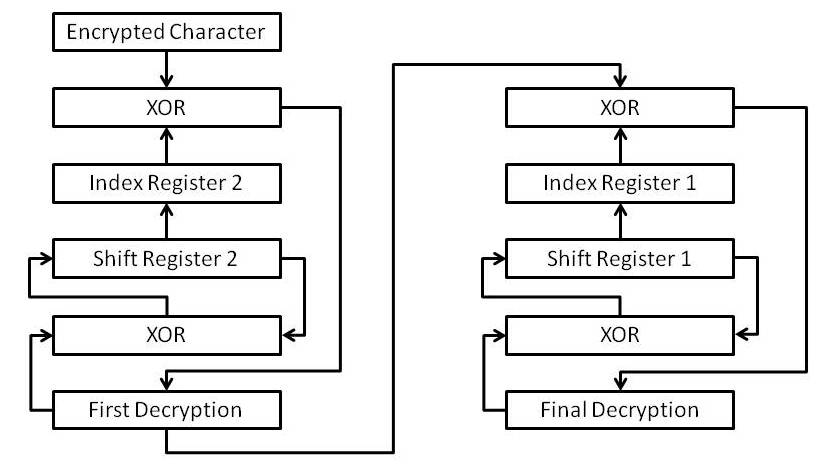# The Decryption Process

The decryption process has to be carried out in reverse to the encryption process.  In Hex Mode, the characters are recovered from the input stream three at a time.  The first two are read as a hexadecimal, single byte pair and the third, a space (ASCII 32) is neglected.  This recovers the original, encrypted ASCII code.  In Stream mode, the characters are recovered one at a time and converted to the ASCII equivalent.  The notation here is the same as that used to describe the encryption process except that for the first stage of decryption, the first decryption is represented by Pi, while, for the second stage, the first decryption is represented by Ci

The decryption process begins by using the XOR function to combine the ASCII value of each cipher text character in turn with the contents of the appropriate element of the index register, indicated by the value of Si :

Pi = Ci XOR R(Si)
5

This produces the deciphered character, Pi.  The XOR function is also used to combine the deciphered character with the contents of Si, the result of which is added to the shift register as the next value of S(256)i+1, after a SHIFT RIGHT LOGICAL operation on the shift register.  The value of Si used for the encryption is overwritten and cannot be recovered:

S (256)i+1 = Pi XOR Si
6

As with encryption, a random number between 0 and 255 is combined with the result of Equation (6)

S (256)i+1 = Pi XOR Si XOR Ri
7

where Ri is the random number.  Because the PRNG is reset to the original seed at the beginning of each encryption and decryption, this will reproduce the same value of S(256)i+1 as that produced during encryption.  The whole encryption process is illustrated by Figure 8.Figure 7:  The decryption process

As each character is decrypted, it is added to the output stream.  Once the decryption is complete, the output file is opened to write the output stream.  The output file has the same file name as the original message.  Because all the original control characters, such as carriage returns and linefeeds were enciphered along with the original text, the output stream can be written as a single record, after which the file is closed.  The plaintext file will be identical to the original message file.